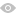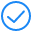## RESOURCES

Current Position: Home>>RESOURCES>>Protocols>>ELISA >>Calculation of ELISA Results

# Calculation of ELISA Results

As the last procedure of ELISA, calculation of results is of great importance to the success of the assay.

1. ELISA samples are always tested in duplicates or in triplicates, then average the absorbance values for each set

of standards and samples.
2. Subtract the average value of zero standard OD (this step is unnecessary in procedure of competitive ELISA).
3. Create standard curve.

Plot a four parameter logistic curve on log-log graph paper, with standard concentration on the x-axis and OD values on the y-axis. The software Origin is recommended to use.

The details are as follows:
Step 1: Input information of the OD value and concentration of the standardsStep 2: Select the curve type:
Select the value box, and then select items in turn as below.Step3: Select the specific X/Y:After setting the above items, click the Fit button, then the curve and its information is produced as showed in step 4.

Step4: Check the information of the standard curve (parameter values, R-Square, etc.)Step5: Set the axis to log-log type.
Double click the Fitted Curve Plot in step 4 to make the curve to editable state. Then double click the X axis and Y axis to set them to log type.Step 6: Calculate the concentration according to the curve
Select the 4th work sheet (Fit NLF Find X from Y1), input or copy the OD value of the sample to the left column, the concentration will be calculated automatically.If the OD value of the sample is out of upper limit of the standard curve in sandwich(or out of the lower limit of competitive ELISA), these samples should be diluted before proceeding with the ELISA to obtain an accurate result. As for these samples, the concentration obtained from the standard curve must be multiplied by the dilution factor when analyzing the results.

### Leave Message

*
*
*
*
*
*
*
Click to load CaptchaMessage submitted successfully !